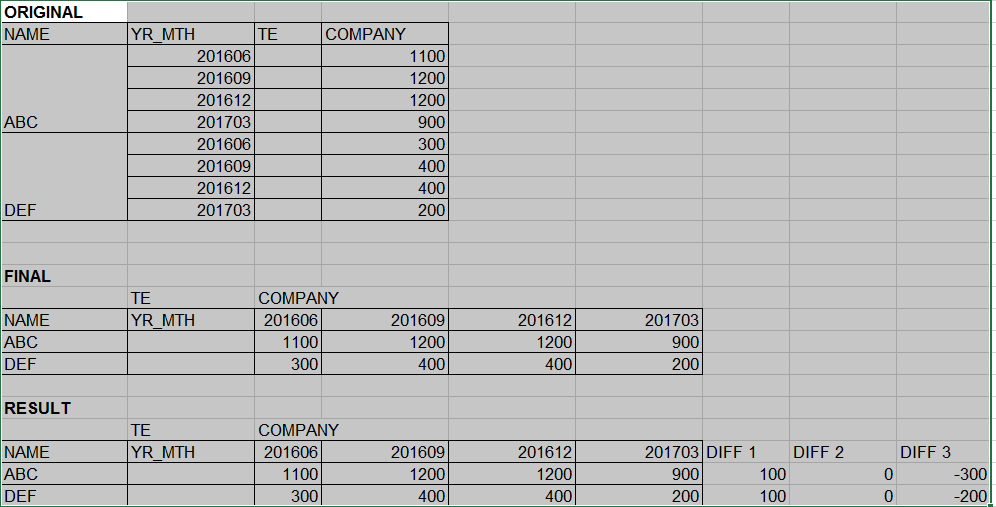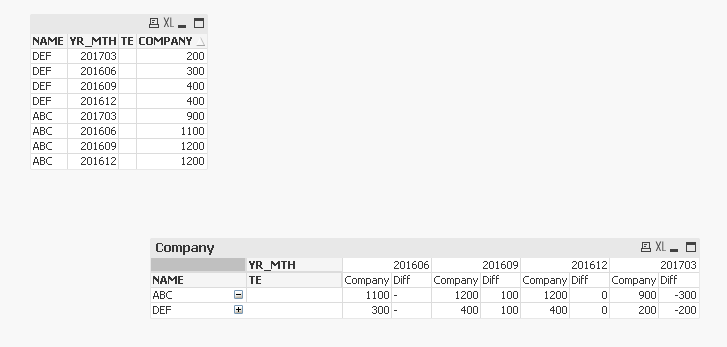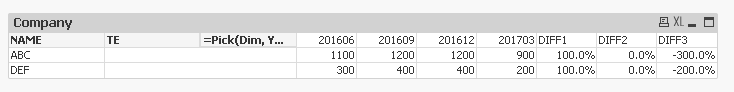# New to QlikView

Discussion board where members can get started with QlikView.

Contributor

## Calculate measures difference in a pivot tableOriginal table (pivot chart) is my source.

Final table is the pivoted version of Original

Result is what's FINAL plus addition of 3 new columns (DIFF1, DIFF2, DIFF3). So I need to calculate
1) difference between 201606 n 201609 for ABC n DEF on column DIFF1
2) difference between 201609 n 201612 for ABC n DEF on column DIFF2

3) difference between 201612 n 201703 for ABC n DEF on column DIFF3.

1 Solution

Accepted SolutionsMVP

## Re: Calculate measures difference in a pivot table

Here check this out17 Replies
HighlightedMVP

## Re: Calculate measures difference in a pivot table

Do you need the exact format where the DIFF columns come after the Quarter columns? Also, will you ever look at only 4 quarter (essentially only 3 difference columns)?MVP

## Re: Calculate measures difference in a pivot table

One option could be to do like thisWhere Diff is an expression like this

Sum(COMPANY)-Before(Sum(COMPANY))

But you won't get the formatting as your image

Contributor

## Re: Calculate measures difference in a pivot table

Yes, I need the exact format. Yes, I'll looking only 4 quarters and 3 difference columns.

I got the output - FINAL. Looking for output - RESULT (is possible, in one chart great; if not may be create a separate chart for DIFF1, DIFF2, DIFF3 and somewhere make it look like one chart)

Contributor

## Re: Calculate measures difference in a pivot table

Thank you Sunny. Unfortunately, this format won't work.MVP

## Re: Calculate measures difference in a pivot table

Here check this outContributor

## Re: Calculate measures difference in a pivot table

Sunny, this is very close to what I need. My TE column looks for different states - NY, CA, FL. So, my output RESULT will be for each state. I'll have 3 charts with RESULT chart - one for NY, one for CA and one for FL.

I do get the COMPANY amount as total value for DIFF1, DIFF2 and DIFF3 as it's adding the individual value for NY, CA and FL. I guess the expression needs to be modified to incorporate the state for DIFF1, DIFF2 and DIFF3 to show correct values at state level rather than summing them up for ABC or DEF. I'm getting error while modifying the expression. Can you provide insight please?MVP

## Re: Calculate measures difference in a pivot table

Not sure I understand... can you share a sample file here?

Contributor

## Re: Calculate measures difference in a pivot table

I slightly tweaked your query to meet my requirement and it's working. Thank you!

Also, the format for DIFF1, DIFF2, DIFF3 columns are in % but all other columns are in \$ format. Since all these numbers are being derived from a single expression, it is possible to have them in different format?MVP

## Re: Calculate measures difference in a pivot table

Sure you can... Use Expression default formatting on the Number's tab... and then use Num to give different formatting for different part of the expressions

Pick(Dim,

Sum(COMPANY),

Num((Sum({<YR_MTH = {"\$(=Min(YR_MTH, 2))"}>} COMPANY) - Sum({<YR_MTH = {"\$(=Min(YR_MTH, 1))"}>} COMPANY))/100, '#.0%'),

Num((Sum({<YR_MTH = {"\$(=Min(YR_MTH, 3))"}>} COMPANY) - Sum({<YR_MTH = {"\$(=Min(YR_MTH, 2))"}>} COMPANY))/100, '#.0%'),

Num((Sum({<YR_MTH = {"\$(=Min(YR_MTH, 4))"}>} COMPANY) - Sum({<YR_MTH = {"\$(=Min(YR_MTH, 3))"}>} COMPANY))/100, '#.0%'))